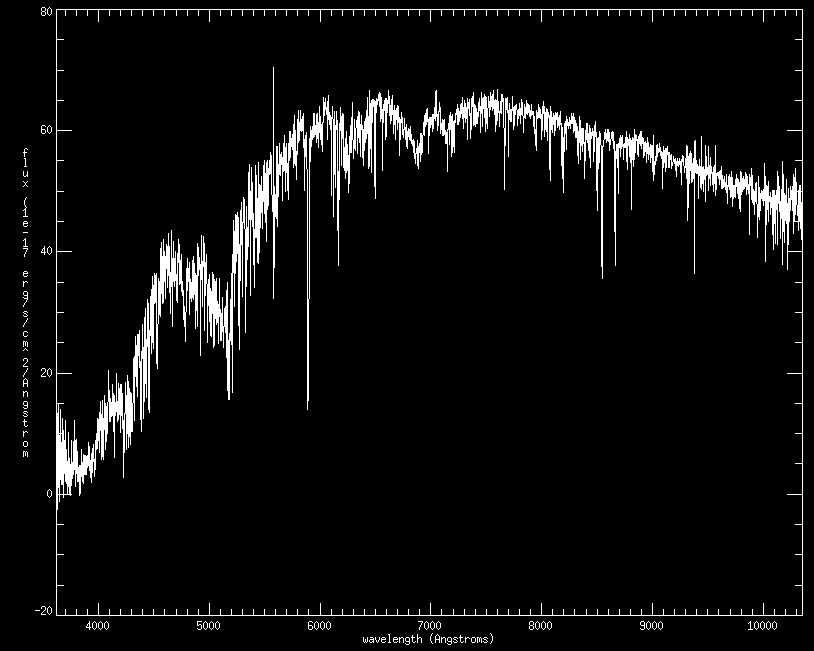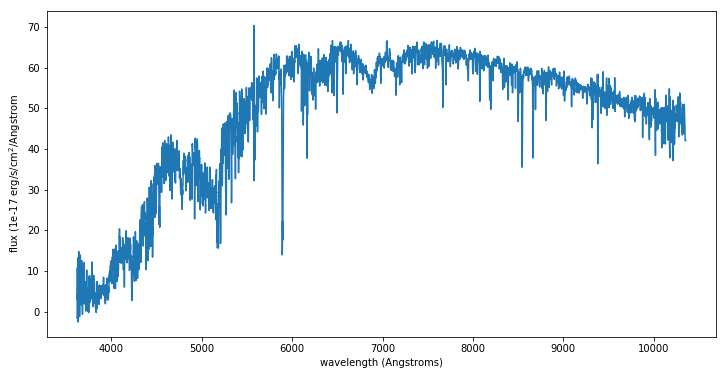# MaStar Tutorials

## IDL Tutorial

This IDL tutorial provides basic examples of how to view the MaStar catalog and spectral data. This tutorial assumes you are using at least IDL 7.1 and have the IDL Astronomy User's Library installed.

### Using the mastarall file

The mastarall file contains important summary info about each star in the catalog. We can load in the catalog with

mastar = mrdfits(dir + 'mastarall-v2_4_3-v1_0_2.fits','GOODSTARS',hdr)


where "dir" is the path to the directory where you've stored the mastarall file. Here, we have read in the GOODSTARS extension. For a full description of the different extensions, see the catalog documentation.

To see the contents of the catalog, type

help,mastar,/str


Let's select all K stars from this catalog with effective temperatures (from input catalog) between 3700 and 5200 K (note not all stars have valid input stellar parameters) and print the number of stars found:

ksel = where(mastar.input_teff ge 3700 and mastar.input_teff lt 5200,count)
print,count


We can use this selector to examine other properties of these stars, such as their surface gravity distribution, e.g.,

plothist,mastar[ksel].input_logg,xtitle='log g'


Or alternatively you can put the K stars into their own IDL structure including all parameters from the mastar structure.

kstars = mastar[ksel]
plothist,kstars.input_logg,xtitle='log g'


### Plotting a MaStar spectrum

Let's plot the spectrum for the first of our K stars selected above. We first need to find its MaNGA ID:

print,kstars.mangaid


Next we will load in the spectra file described here:

goodspec = mrdfits(dir+"mastar-goodspec-v2_4_3-v1_0_2.fits.gz",1,hdr2)


Cross-reference the MaNGA ID from the mastarall file with the goodspec file to isolate the spectra we're interested in. In this case, the star was observed multiple times, so we will plot the first visit:

visits = where(strtrim(goodspec.MANGAID,2) eq strtrim(kstars.MANGAID,2))
wl = goodspec[visits].WAVE
flux = goodspec[visits].FLUX


Now let's plot the spectrum:

plot,wl,flux,xtitle='wavelength (Angstroms)',ytitle='flux (1e-17 erg/s/cm^2/Angstrom',/xsty,font=0The spectrum for one MaStar K star

## Python Tutorial

This Python tutorial provides basic examples of how to view the MaStar catalog and spectral data. This tutorial assumes you are using at least Python 2.7 and have the Astropy package installed.

### Using the mastarall file

First, import the necessary packages:

import astropy.io.fits as fits
import numpy as np
import matplotlib.pyplot as plt


The mastarall file contains important summary info about each star in the catalog. We can load in the catalog with

mastarall = fits.open(dir + 'mastarall-v2_4_3-v1_0_2.fits')


where “dir” is the path to the directory where you’ve stored the mastarall file.

To look at the header information of the fits file and identify which extension to use, type

mastarall.info()


We are interested in the "GOODSTARS" extension, so we will be using extension 1. For a full description of the different extensions, see the catalog documentation.

To learn what information this extension contains, list all of the column names using:

mastarall.data.names


Let’s select all K stars from this catalog with effective temperatures (from input catalog) between 3700 and 5200K (note not all stars have valid input stellar parameters) and print the number of stars found:

ksel = np.logical_and(mastarall.data["INPUT_TEFF"] > 3700 , mastarall.data["INPUT_TEFF"] < 5200)
print(len(mastarall.data[ksel]))


We can use this selector to examine other properties of these stars, such as their surface gravity distribution, e.g.,

plt.hist(mastarall.data[ksel]["INPUT_LOGG"])
plt.title("log g")
plt.show()


Or alternatively you can put the K stars into their own structure including all parameters from the mastarall structure.

kstars = mastarall.data[ksel]
plt.hist(kstars["INPUT_LOGG"])
plt.title("log g")
plt.show()


### Plotting a MaStar spectrum

Let’s plot the spectrum for the first of our K stars selected above. We first need to find its MaNGA ID:

print(kstars["MANGAID"])


Next we will load in the spectra file described here:

goodspec = fits.open("mastar-goodspec-v2_4_3-v1_0_2.fits.gz").data


Cross-reference the MaNGA ID from the mastarall file with the goodspec file to isolate the spectra we're interested in. In this case, the star was observed multiple times, so we will plot the first visit:

visits = goodspec["MANGAID"] == kstars["MANGAID"]
wl = goodspec[visits]["WAVE"]
flux = goodspec[visits]["FLUX"]


Now let’s plot the spectrum:

plt.plot(wl,flux)
plt.ylabel("flux (1e-17 erg/s/cm$^2$/Angstrom")
plt.xlabel("wavelength (Angstroms)")
plt.show()The spectrum for one MaStar K star